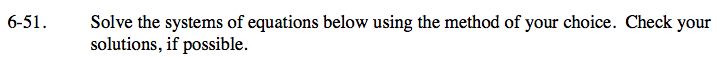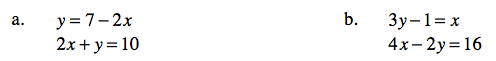### Home > CAAC > Chapter 6 > Lesson 6.2.2 > Problem6-51

6-51.
1. Solve the systems of equations below using the method of your choice. Check your solutions, if possible. Homework Help ✎

1. y = 7 − 2x
2x + y = 10

2. 3y − 1 = x
4x − 2y = 16Substitute 7 − 2x for y.

2x + (7 − 2x) = 10

Combine like terms.

7 = 10

There is no solution.

Substitute 3y − 1 in for x.

Follow the steps in part (a).